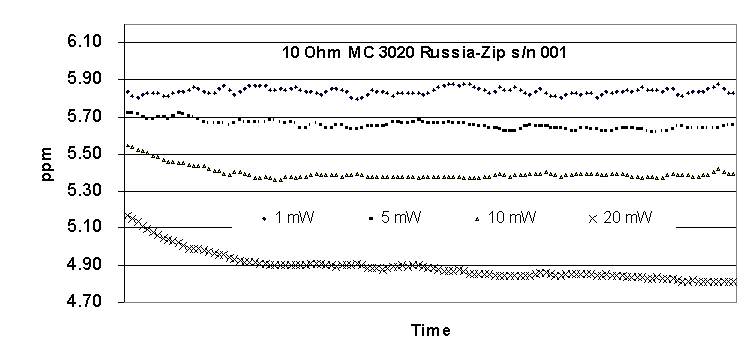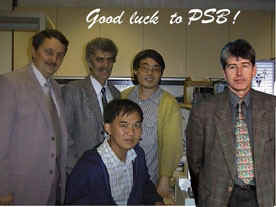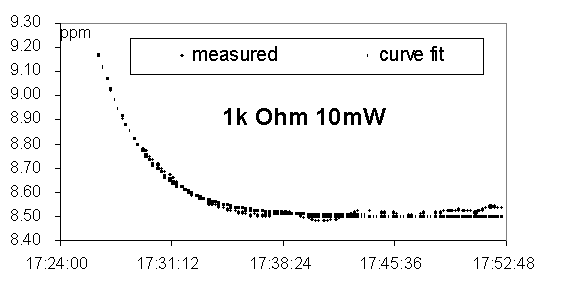ЦЕНЫ

1.  Статья "Мощностные характеристики метолло-фольговых мер сопротивления", "Power Dependence Characteristics of Metal Foil Type Standard Resistors" (ЗИП-Научприбор, фирма Юримов, центр метрологии Юго-восточной Азии г.Сингапур).

V. Zaslavsky (В.А. Заславский), ZIP-Nauchpribor, Russia
N. Daniltchenko, YURIMOV Ltd., Russia
Sim Tian Yeong  Productivity and Standards Board, SingaporeAbstract

This paper reported the investigation results of the power dependence characteristics of metal foil type standard resistors. The power coefficient and heating temperature time constant were measured for 10 Ohm, 100 Ohm and 1 kOhm metal foil type standard resistors.

Introduction

When the very precision measurements of the resistance standards, it is needed to know how supplied power changes the results of measurement . This paper reported the investigation results of the power characteristics of the metal foil type standard resistors.

The Power Coefficient Determination

Three standard resistors, 10 Ohm, 100 Ohm and 1000 Ohm with nominal applied power of 10 mW were used in the experiment. These standard resistors were put into an oil bath with temperature controlled at 30oC and the temperature deviation of the oil bath is about ±2.5moC.The measurements were carried out held at PSB, Singapore using the MI 6010A Automatic DC Resistance Bridge with uncertainty less than 0.2 ppm.

Every resistance standard was tested under applied power of 1 mW, 5 mW, 10 mW and 20 mW. The 20 mW power was used only for this experiment and is not intended to use these standard resistors with higher power from the nominal applied power.

The measurements were delay for about 13 seconds from the moment of supplying power and 120 data were taken. 10 minutes of interval was given between different applied power after a complete cycle of measurements.

Figure 1 shows the measurement results of a 10 Ohm standard rsistor the measurement. The 100 Ohm and 1 kOhm standard resistors have a similar characteristic.

The 10 mW applied power was taken as reference and all measurements taken at different applied power were corrected with reference to this value. In practice, any other power up to 10 mW could provide reliable measurements and can be taken as reference point. The deviations of resistance from the 10mW are summarised in Figure 2.It can be seen from Figure 2 that the metal foil standard resistors have a linear power characteristic for power below 20 mW and within this power range the correction for the resistance at other power different from the reference power can be estimated. The power coefficient (Kp) which is defined as deviation (microOhm/Ohm) per applied power (mW) is given by the slope of Figure 2. Table 2 summarised the power coefficients for the metal foil standard resistors.

The Heating Time Constant

Another characteristic of these standard resistors

could be observed from the Figure 1 is the resistance changes exponantially when higher power is added. The heating time constant according to the changing of resistance value under an applied power can be estimated as shown in Figure 3, the time constant for standard resistor 1000 Ohm at 10 mW power is about 3 mintues.

Table 3 A summary of power coefficients

 Standard Resistor (Ohm) Power Coefficient Kp (microOhm/Ohm/mW) 10 -0.06 100 -0.026 1000 -0.148

Figure. 3 Heating effect of the standard resistor

As shown in Figure 3, It is important to avoid taking measurement at the transitional period. To decrease this mistake the measurement could be started at about 3t delay time after the moment of supplying power. In this case, 3t » 10 minutes.

Conclusion

The metal foil type standard resistors used in this experiment shown linear power characteristc. This characteristic will allowed user to add correction for measurement which is done with different applied power. The study of thermal time constant is also allowed user to avoid taking measurement at the transient period as measurement data taken at this period will affect the mean value of the measurement. More results will be presented at the Conference.Acknowledgments

The authors wish to express their gratitude to Mr. Ang Chee Kiang and Mr Eddie Tan of the PSB National Measurement Centre, Singapore for helping in set up of measurements of the resistance standards.

Reference

 R. E. Elmquist and R.F.Dziuba, “Loading Effects Resistance Scaling”, Conference Digest of CPEM’96, pp.334-335, Braunschweig Germany, June 17-20, 1996.